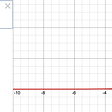# Why Study this?

This form of analysis is relatively easy to explain and it is a good way for students to start thinking about analyzing data.

# What will we learn?

We will look at mathematical examples and use real world analogies for apply the concept of dissimilarity calculations for binary and asymmetric binary attributes. Basic examples will be provided. Then there will be challenge exercises to help you solidify your knowledge.

# What is a binary attribute?

A symmetric binary attribute is a nominal attribute with only two categories of 0 or 1. A binary attribute is symmetric. The two categorical values have the same significance. For example, gender could have values of male or female. The Morgan Kaufmann Series in Data Management Systems defines a binary attribute as an attribute that has only one of two states: 0 and 1, where 0 means that the attribute is absent, and 1 means that it is present.

# How do we calculate the dissimilarity for the binary attributes in the dataset shown below?

The dissimilarity formula for binary attributes is:

The first step is to build the contingency table for a given dataset.

1. For attribute A1 we increment 0->1
2. For attribute A2 we increment 1->1
3. For attribute A3 we increment 0->0

The next step is to evaluate the dissimilarity formula

# How do we calculate the dissimilarity for the asymmetric binary attributes in the dataset shown below?

The dissimilarity formula for asymmetric binary attributes is:

An asymmetric binary attribute means the value of 1 is more important than 0. An example of this could be the value of light or dark. If an area is slightly brighter than an area then the significance is higher.

Challenge:

The contingency table for object x1 and x2 evaluates to:

The dissimilarity formula for asymmetric binary attributes is:

There is no need to determine the value for element d in the contingency table.

# Given the dataset below, what is the dissimilarity between objects x1 and x2?

d(x1,x2) = 1+1/(1+1+0+0) = 1

# What would be the dissimilarity if the attributes were asymmetric binary?

d(x1,x2) = 1+1/(1+1+0) = 1

# Conclusion

If you enjoyed these exercises and analogies then I suggest that you try creating your own examples. The process of developing your own material will help you develop an intuition for these concepts. This is supposed to be fun!

# References

Jian Pei Jiawei Han, Micheline Kamber.Data Mining. Morgan Kaufman.

Ray-Tyler Hashemi Yamcraw Professor of Engineering, Data Mining, Georgia Southern University

--

--

--

## More from MLearning.ai

Data Scientists must think like an artist when finding a solution when creating a piece of code. ⚪️ Artists enjoy working on interesting problems, even if there is no obvious answer ⚪️ linktr.ee/mlearning 🔵 Follow to join our 18K+ Unique DAILY Readers 🟠

## The Math behind Schrödinger Equation: The Wave-particle duality and the Heat equation.## Exploring The Infinite With Primecoin## Maximum Sum Subarray Problem## Frege on Numbers, or ‘135664 Fingers’## Poisson Distribution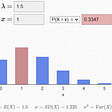## A New Exponential number Algorithm that uses Binary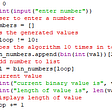## Finding Order in Chaos## Why there is something rather than nothing da?## Evan Gertis

I like building technology. That’s pretty much what I live for. http://www.evan-gertis.com/

## How to use Cosine Similarity and the Tanimoto Coefficient## Ames Housing Price Predic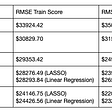## How To Classify Handwritten Digits Using A Multilayer Perceptron Classifier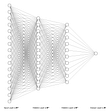## Logistics Regression: How does it work